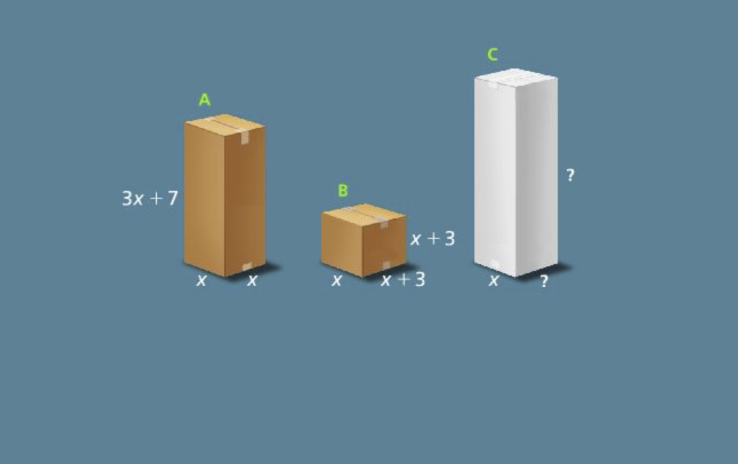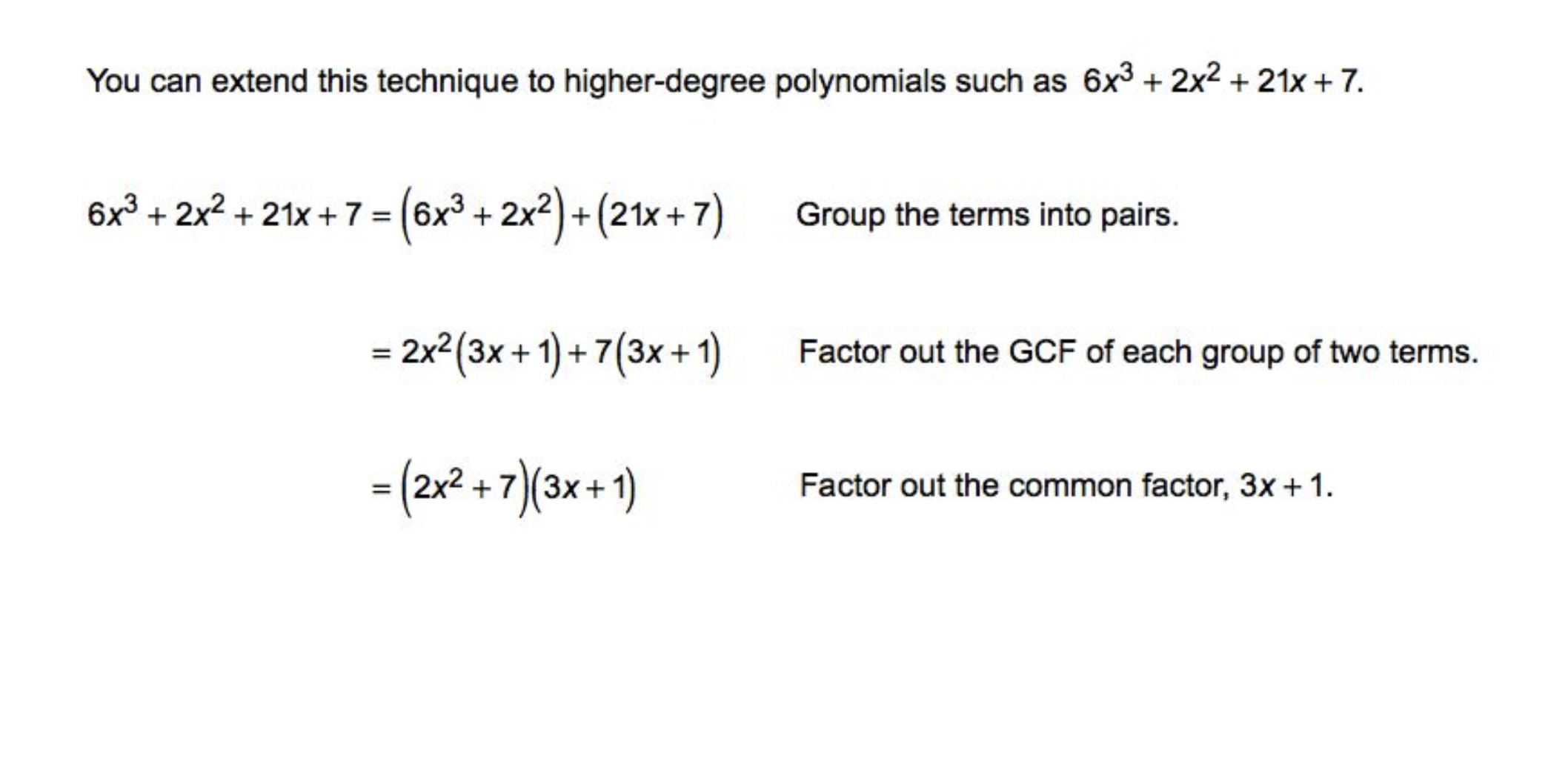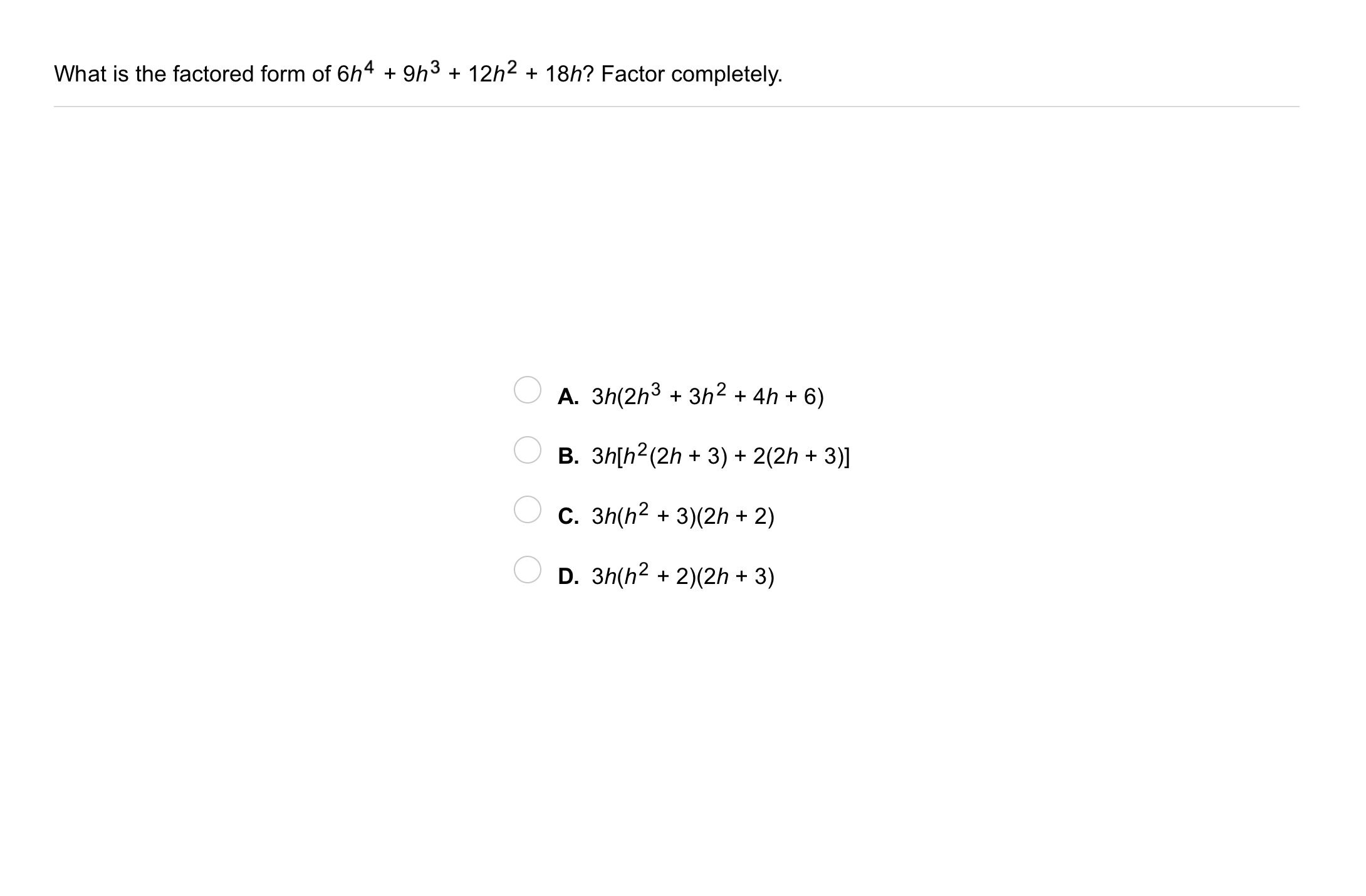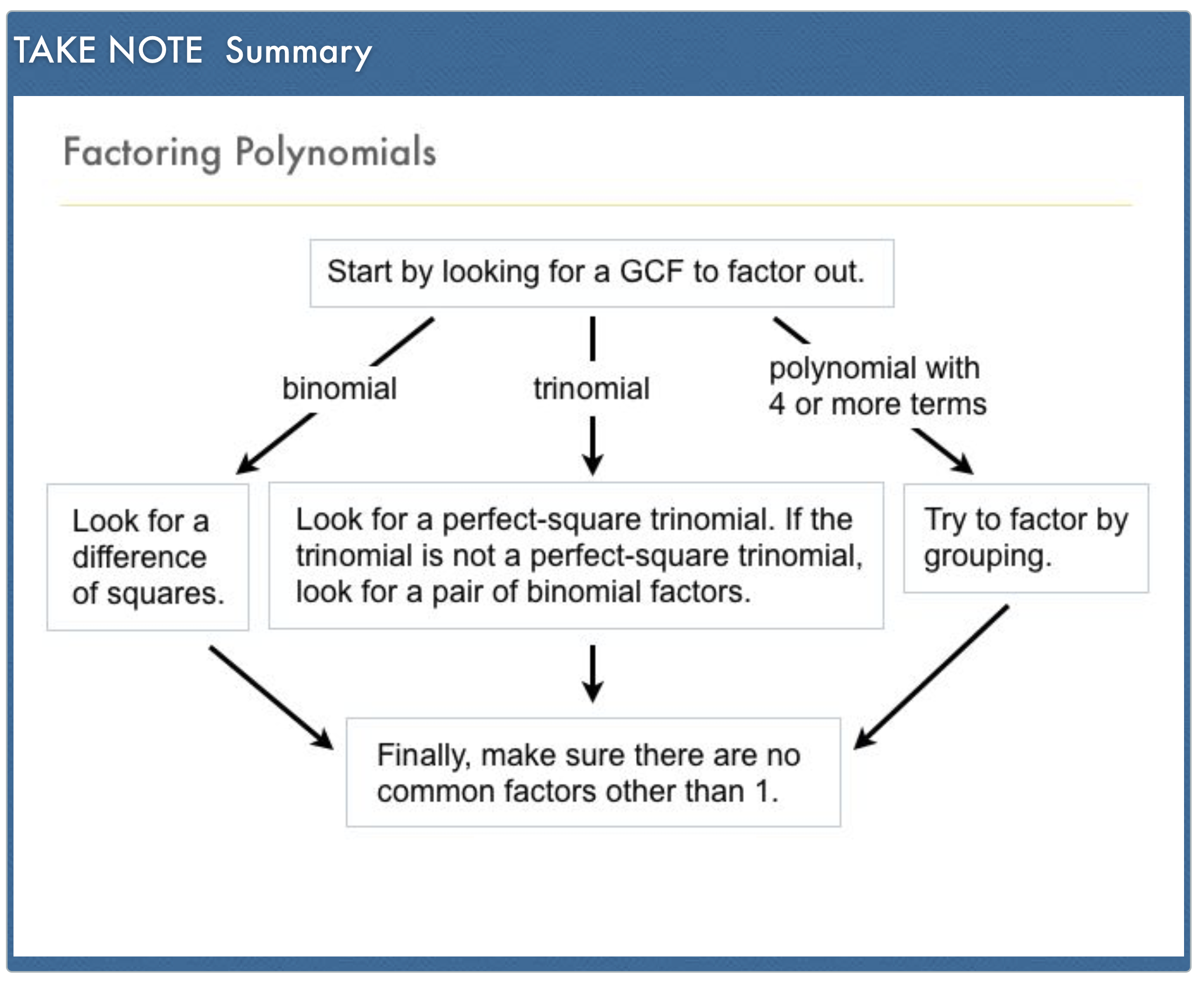Algebra 1 8-8 Guided Practice: Factoring by Grouping
starstarstarstarstarstarstarstarstarstar
by Matthew Richardson
| 4 Questions1
1
10
Solve It! A packaging company sells two kinds of boxes, Box A and Box B. The company is designing a new box, Box C, that will have the same volume as Boxes A and B combined. Suppose one dimension of Box C is x. What could be the other two dimensions? Select all that apply.
4x + 9
x + 9
4x + 1
x + 12
10
Problem 1 Got It? What is the factored form of the polynomial?3
3
10
Problem 2 Got It?
A
B
C
D
4
10
Problem 3 Got It: Geometry: A rectangular prism has volume represented by the trinomial below.
Which three expressions below could together represent the dimensions of the prism? Use factoring. Select all that apply.
6x + 1
2x
x
5x + 2